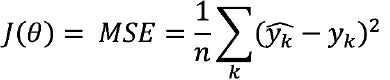# Tuning a Machine Learning Model

## Loss Functions

A loss function serves as the objective function that the AI/ML algorithm is seeking to optimize during training efforts, and is often represented as a function of model weights, J(θ). During model training, the AI/ML algorithm aims to minimize the loss function. Data scientists often consider different loss functions to improve the model – e.g., make the model less sensitive to outliers, better handle noise, or reduce overfitting.

A simple example of a loss function is mean squared error (MSE), which often is used to optimize regression models. MSE measures the average of squared difference between predictions and actual output values. The equation for a loss function using MSE can be written as follows:Whererepresents a model prediction,yk represents an actual value, and there are n data points.

It is important, however, to recognize the weaknesses of loss functions. Over-relying on loss functions as an indicator of prediction accuracy may lead to erroneous model setpoints. For example, the two linear regression models shown in the following figure have the same MSE, but the model on the left is under-predicting while the model on the right is over-predicting.

Loss Function is Insufficient as Only Evaluation MetricFigure 13 These two linear regression models have the same MSE, but the model on the left is under-predicting and the model on the right is over-predicting.